All submissions of the EM system will be redirected to Online Manuscript Submission System. Authors are requested to submit articles directly to Online Manuscript Submission System of respective journal.

# Original Article

, Volume: 11( 2) DOI: 10.37532/2320-6756.2023.11(2).321

## Exploration and Research of Meta-system Space Based on the Thought of Relative Criterion

*Correspondence:
Jingang Liu
Professor and Doctoral supervisor, Capital Normal University, China
E-mail:
liujg2000@163.com

Received date: 30-January-2023, Manuscript No. tspa-23-88180; Editor assigned: 02-February-2023, PreQC No. tspa-23-88180 (PQ); Reviewed: 06-February-2023, QC No. tspa-23-88180 (Q); Revised: 07-February-2023, Manuscript No. tspa-23-88180 (R); Published: 21- February-2023, DOI. 10.37532/2320-6756.2023.11(2).321

Citation: Liu J. Exploration and Research of Meta-system Space Based on the Thought of Relative Criterion.2023;11(2):321.

### Abstract

In this paper, based on the thought of relative criterion, a space system of continuous quantities is created, which is a mathematical convergence system, called a meta- system. It is expounded that the meta-system consists of a binary star at the center and a galaxy orbiting the binary star at the same angular velocity, and it is a relatively static cluster of dynamically balanced stars in the universe. Based on the mathematical model of the meta-system, this paper deduces the theoretical formula of the relatively meta-system space through the relative criterion formula of the meta-system theory, unifying the four forces acting on the planet. Based on the formula of the meta-system space theory of the relative criterion, four conclusions of the meta-system space are deduced, and it is found that each galaxy is affected by the central binary star of the meta-system. Based on the space of meta- system, the objective laws of the planets rotating around the sun in the same plane and direction are revealed. This paper also provides a new cognition for exploring and observing the central binary star around which the sun revolves.

### Keywords

Relative criterion; Continuous quantity; Meta-system; Space.

### Introduction

Meta-system space refers to the physical space of a certain group of celestial bodies in the universe, with a system space consisting of a center and a clear range; the galaxies in the system are no longer changing and are in a dynamic and stable state. In the study of relative criterion of discrete quantity system, the system composed of something has the mathematical convergence solution of relative criterion about meta-system. Through the relative criterion formula :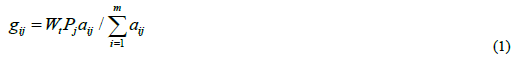Model 2 is calculated from model 1, as shown in FIG. 1; after model 3 is calculated from model 2 through equation 1, all models after model 4, 5, 6... are the same as model 2, as shown in FIG. 2. In the discrete system, model 2 is defined as a relative criterion meta-system . There definite be a relative criterion meta-system in the continuous quantity system either.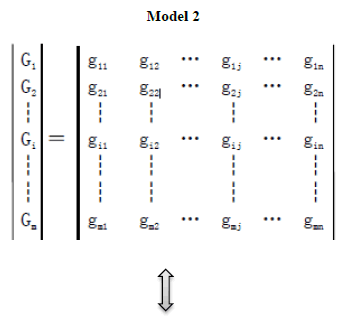Figure 2: Evolution and conservation of meta-system.

If the continuous quantity system is aimed at 2 space, then there also certain be a relative criterion meta-system with stable and constant dynamic balance in this space. For example, Model 2:

To analyze and study the problems of objective things with the thought of relative criterion, there must be a group of things and the scope of the group, that is, a system. Similarly, to analyze and study the space problem of continuous quantity, there must be a group of stars and a system with a clear range. Metasystem space is a kind of dynamic balance space that no longer changes, such as the space where the solar system is located. Because the sun and its planets move very regularly and are in a stable state, it is a relative criterion metasystem that will not change in relative criterion theory. The stars clusters with the same operation state have a clear range. As long as there is no influence from outside the system, the meta-system will not change in theory, namely:

As shown in Figure 2 . According to equation 1: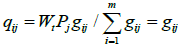The sum of the relative quantities of the elements in the meta-system is a constant WtPj, which means that the meta-system space will not change again. Through the study of discrete quantity system, we get a one-time convergent meta-system, and believe that the universe is a space composed of dynamically balanced and stable meta-systems and non-meta systems. Some non-meta systems be a meta-system formed in the process of continuous development and change, which has evolved into a dynamic and stable state without development and change. Some mix with other celestial bodies and evolve into others.

### The Scope and Center of Meta-system Space

Meta-system is a system with mathematical convergence and a dynamic system with stable changes. Therefore, in the space of meta-system, there is no celestial bodies at rest or moving at a uniform speed in a straight line. In the meta3 system, if the celestial bodies is stationary, the system elements expressed in mathematics are all zero. If the celestial bodies moves in a uniform straight line, the mathematical representation of the system element is a constant, which is not a dynamic equilibrium system. If there are celestial bodies moving in a straight line, the system space has no boundary and is not a meta-system space. Meta-system space is simply a basic unit of the universe, which has both a center and a range, and is not boundless. If the system does not determine the specific scope, there will be no exact relative criterion conclusion. Galaxies with the same operating state constitute a meta-system space. For example, galaxies with the same running state as the sun or relatively static with the sun constitute a meta-system space. As shown in FIG. 3, there are multiple meta-system spaces, and each galaxy in each meta-system is running around the center at the same angular velocity.

Because the angular velocities of the galaxies in the meta-system space are the same, the galaxies are relatively stationary. The space where all relatively static galaxies are located is the space scope of a meta-system.

Since there are no static or uniformly rectilinear moving celestial bodies in the meta-system, the center of the meta-system will not be a single celestial body. A single celestial body, no matter how large it is, is not the center of the meta-system, but the center is two mutually revolving binary star. As shown in FIG. 4:

A single celestial body, such as the sun, is the center of the solar system, but not the center of the meta-system space. The sun is not stationary, nor is it moving at a uniform speed in a straight line, and its running state is stable, so it be moving at a uniform speed in a circle. A celestial body moving along circle, regardless of its size, is moving around its center, not its own center. The center of the meta-system will not be three or more mutually revolving celestial bodies. There is synchronization problem when more than 3 celestial bodies rotate. Once the multiple celestial bodies rotate synchronously, they are relatively stationary. Relatively stationary multiple celestial bodies will eventually become two mutually rotating or a spinning celestial body under the action of gravitation. Under the action of the two celestial bodies revolving around each other, they drive other celestial bodies around which the force is applied to rotate together, until all the celestial bodies under the action and the central binary star rotate in the same plane and reach the same angular velocity, a stable dynamic equilibrium meta-system is realized.

The feature of binary star in which the center of the meta-system revolves around each other is that there are no other celestial bodies between the binary star. All celestial bodies in the metasystem are in dynamic equilibrium and have the same angular velocity, so they are relatively stationary. Under the gravitational action of the central binary star of the meta-system, the surrounding celestial bodies evolve from a spherical group to a cylindrical one, and finally become a plane disc. In the process of evolution, these celestial bodies are either swallowed by binary star or pulled to circle around the plane of rotation until the celestial bodies without asynchronous operation are relatively static and evolve to the ultimate, that is, meta-system space. The force exerted by the central binary of the meta-system on the surrounding celestial bodies is the superposition of repulsive force and gravitational force. With the increase of distance, the repulsive force decays faster than the gravitational force. In a large range, the gravity of the celestial bodies by the central binary star increases with the increase of distance. Relative to the space scale of the metasystem, the distance between the central rotating binaries is very close, but the binaries can be distinguished by observation.

### Meta-system Space Mathematics Model

The celestial bodies in the meta-system space are not only affected by the central binary star force; it is also affected by the interaction between celestial bodies. The spin force of the celestial body itself; and its own planet or satellite. These four forces gather many celestial bodies together, but their volume, mass, energy and other states are different, while each celestial body can operate accurately and orderly. Without the influence of the alien bodies from the meta-system, the galaxies will run stably forever. The conservation of energy and mass will become eternal and stable.

All galaxies in the meta-system are relatively stationary, although they are in motion and have different accelerations. It can’t be seen that the binary star are circling in any galaxy. The distance between galaxies is fixed, and the number of galaxies is also constant. These characteristics are similar to discrete quantity systems. The creation of the spatial model of the meta-system can refer to the relative criterion meta-system model, as shown in FIG. 5.

The meta-system is composed of stable galaxies, and its evolution has reached a dynamic balance, which is mathematically convergent. According to equation 2, it is obtained that: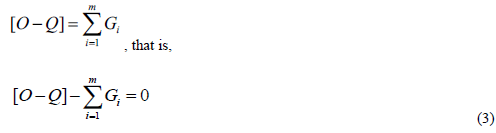Where [O-Q] is the energy or force of the binary stars, and Gi is the energy or force of each galaxy. There are m galaxies in the whole meta-system. Their energy and mass do not increase or decrease. The force and reaction between each galaxy and the central binary star are equal, and their total or combined force is 0. The mathematical model for each galaxy Gi is shown in FIG. 6:

Since the state of each star in the galaxy is constant and mathematically convergent, according to equation 2, we can get:In equation 4, Si is the star of galaxy i, and star Si has k planets in total. ghj is the jth aspect characteristic quantity of planet h, and Pj is the objective proportion of jth aspect characteristic quantity to other quantities. Substitute equation 3 into equation 4 to get:That is, Gi>0; Galaxy Gi has energy or force from binary star [O-Q]. The meta-system is balanced, and the balance of the galaxy Gi has energy input, indicating that the galaxy is an energy consuming system. In a dynamic balance system, the dynamic galaxy needs energy supply to achieve balance while consuming energy. Based on the law of universal gravitation, if there are two stars Si in a galaxy Gi, the galaxy is unstable . If there are two or more stars Si in a galaxy Gi, when they rotate synchronously with the central binary star, they must be in the same orbital region, and finally become one due to the mutual gravity between stars. Therefore, a galaxy Gi can only have one star Si in the orbital orbit around the binary star, but there may be different numbers of planets ghj. The metasystem is composed of galaxy Gi, and each galaxy Gi is composed of three grade, the star Si -planet ghj -satellite qhj. The eight planets in the solar system are different in size, composition, and state and operation law. Each galaxy Gi has different energy levels and is not in the same orbit, so the celestial body ghj in different galaxies Gi is even more dissimilar.

### Solar System in Meta-system Space

FIG. 5 of the mathematical model of the metasystem is composed of galaxy Gi. The most familiar galaxy is the solar system, as shown in FIG. 7.

In the galaxy model in FIG. 6, that is, from the sun, the orbits of the eight planets revolving around the sun are all round. There is no scientific explanation for why all planets move in the same direction and on the same plane around the sun. If these planets come from the explosion of the sun, then the orbit of the planets should be three-dimensional and multiple directional, not to mention in the same plane. The planets produced by the solar explosion cannot explain the orbits of these planets. Because of the central binary star circling effect in the space of the meta-system, when the planets are brought to the orbit of star with the same energy level to run synchronously with the star, they are attracted by the star and become planets that run around the star. The moon is a satellite attracted by the earth to become the earth. When people look at the moon on the earth, its running track is round, but when they look at the moon on the solar system, it is a sine curve of swing, as shown in FIG. 8.

Viewing the solar system from the perspective of meta-system space is the circular motion of the sun around the central binary star [O-Q] of the meta-system space; in the meta-system, the orbits of planets revolving around the sun are not circular. According to the existing published data of the solar system, there are at least 8 planets orbiting the sun, swinging at different frequencies and amplitudes, taking the sun's track as the center, and doing sinusoidal motions, as shown in FIG. 9.

At present, only the revolution speed of the sun is known, but the revolution period and radius length are unknown, because the central binary star [O-Q] around which the sun rotates is not observed or not found.

The total mass of all planets revolving around the sun is less than 0.2% of the sun's mass. From the perspective of meta-system space, these planets have little influence on the solar system. However, the change of any planet will cause the solar system to reach a new dynamic balance. Because the mass of the planet is far less than the sun, the planet must move faster than the sun to be in the same energy level orbit with the sun. The speed of each planet relative to the sun be superimposed with the speed of the sun's motion, that is, the action of the central binary star on the planet. They move around the binary star in center of the meta-system with the sun on a circumference, and at the same time, the planets swing in a sinusoidal curve under the action of the sun's gravity.

Since the angular velocity of the galaxy Gi around the central binary star is the same, Therefore, the faster the speed of the star and the higher the energy level, the farther it is from the binary star. Planets that move faster than the sun are on the outside of the sun's orbit. If the planet is attracted by the sun at the outer side, for example, Jupiter A will change its running direction and move towards the sun, and enter the inner measurement of the sun's running orbit in front of the sun's running, as shown in the FIG. 10.

On the outer side of the sun's orbit, the planet along the sun's direction of motion, it's the speed is the sum with the sun's orbit speed; On the inner side of the solar track, the slowest speed is the subtract between the speed of sun and the planet, but planets moves in a uniform circle relative to the sun. On the inner side of the sun's orbit, for example, the speed of Planet B is slower than that of the sun, if it is attracted by the sun, the speed of Planet B will accelerate, but not exceed the sun, but enter the sun's embrace . This makes it clear why the planets rotate in the same direction around the sun, and also in the same which sun's revolution direction. The earth on which we live has little influence on the meta-system space and the solar system, but the influence of the metasystem and the solar system on the earth is huge. According to equation 5: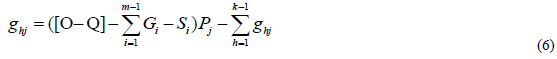According to equation 6, the earth ghj is affected by the four forces (or energies) of the binary star [O-Q] in the center of the metasystem, other galaxies ΣGi, solar Si, other planets and satellites Σghj. The first three and the fourth of the four forces have a multiple relationship of Pj, and the fourth force is fluctuating (as shown in FIG. 9). The four forces acting on the planet are unified in the theoretical equation 6 derived from the mathematical model established by the relative criterion ideological theory .

### Conclusion

Based on the mathematical model established for the meta-system based on the thought of relative criterion, following the law of universal gravitation, through theoretical analysis and deduction, four conclusions of the meta-system space are deduced from the equations 3, 4, 5 and 6 obtained:

• The meta-system space is centered on the revolving binary star [O-Q], and a single star can’t be the center of the metasystem space.

•The angular velocity of each galaxy Gi is the same as that of the binary star [O-Q], and it is in the same plane.

•All celestial bodies are subject to external forces, and they move in curves. The celestial bodies without uniform linearmotion or static; there are no two celestial bodies with the same motion state in the same meta-system space.

•The dynamic balance of galaxy Gi is supplied by the energy of the central binary star on metasystem space.

There are many planets of similar size to Earth in the meta-system. According to inference 3 of the meta-system, there are no celestial body in the same state in the same meta-system. That is to say, no planet with the same state as the Earth can be found in the space of the same metasystem.

At present, the central binary star around which the sun revolves is unknown, but the central binary star [O-Q] of the meta-system is the center around which the sun Si revolves. According to the meta-system inference 1 and 2, the binary star [O-Q] observed in the solar system should be two stars with a certain distance and no celestial body in the middle is relatively stationary. After recognizing that the center of the sun's orbit is binary star, the central binary star [O-Q] around which the solar system revolves can be observed on the earth through efforts. The theoretical formulas and corollaries in this paper can be confirmed when the binary star [O-Q] around which the sun revolves are observed.

Space is continuous. In a meta-system, even if the same object relative different spaces, its trajectory and state are different. Analyzing and studying anything can’t be separated from the space where things are. Schrodinger's Cat explained this kind view on space, the closed and open boxes are two kinds of spaces, and different spaces will lead to different conclusions. In the meta-system, each celestial body occupies different spatial positions, and has different characteristics. Based on the idea of relative criterion, it is determined that the meta-system is a stable and dynamically balanced space, and the unified equation 6 that planets are subjected to four kinds of forces is obtained. According to the theoretical equation 5, the energy consumption of galaxy Gi in the meta-system is unbalanced, and it is found that it is an energy consuming system. This conclusion makes us realize that the balance of the solar system is affected by the binary stars in the center meta-system. If there is no reference coordinate, there will be no speed of the sun moves. If the sun moves in a uniform straight line, no matter what the speed is, it is still. The sun is 300000 times the mass of the earth, and the circular motion of the sun at high speed has huge acceleration, which proves that the force from the center binary (OQ) of the meta-system is very strong, even exceeding the force of the sun on the earth.

When the limit of speed is considered to be the speed of light and all inertial systems are applicable, "relativity" makes our understanding of nature deeper and closer to the truth. When a part of the universe is a metasystem space, the idea of relative criterion makes our understanding of nature clearer and simpler. Let us clearly understand why the planets around the sun are in the same plane and rotate in the same direction; the center of the meta-system space is a pair of mutually rotating stars. There is no criterion without relativity. In a stable dynamic meta-system, the relationship between space and time and the time standard for subjective determination need further exploration and research.

### Acknowledgment

Thank you in advance for the observers who observed the central binary star around which the sun revolves. We are looking forward to observing the binary star in the center of the meta-system Space, to confirm the correctness of the cognition universe based on thought of relative criterion.

### References

Welcome Message

Select your language of interest to view the total content in your interested language

###### Citations : 272

Journal of Physics & Astronomy received 272 citations as per Google Scholar report

#### Indexed In

• Open J Gate
• China National Knowledge Infrastructure (CNKI)
• Cosmos IF
• Directory of Research Journal Indexing (DRJI)
• MIAR
• Secret Search Engine Labs
• Euro Pub
• clarivate-web-of-science-logo-vector
• ICMJE

View More

### 9th International Conference on Physics

Edinburgh, Scotland

### 6th International Conference on Astronomy, Astrophysics and Space Science

Budapest, Hungary

Berlin, Germany

### 9th International Conference on Physics

Edinburgh, Scotland

### 6th International Conference on Astronomy, Astrophysics and Space Science

Budapest, Hungary

### 10th International Conference on Theoretical and Applied Physics